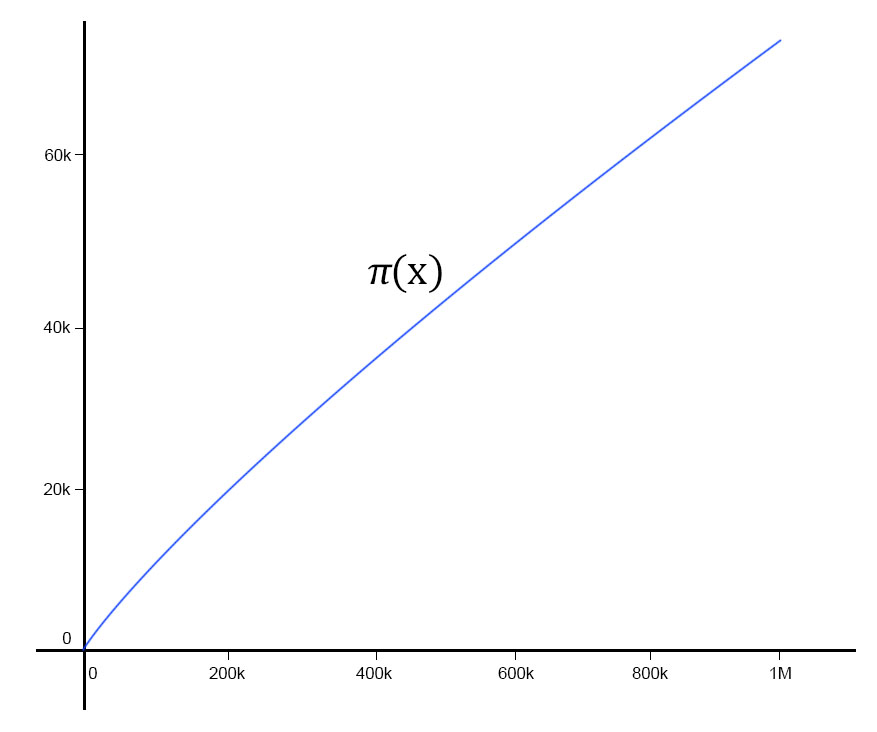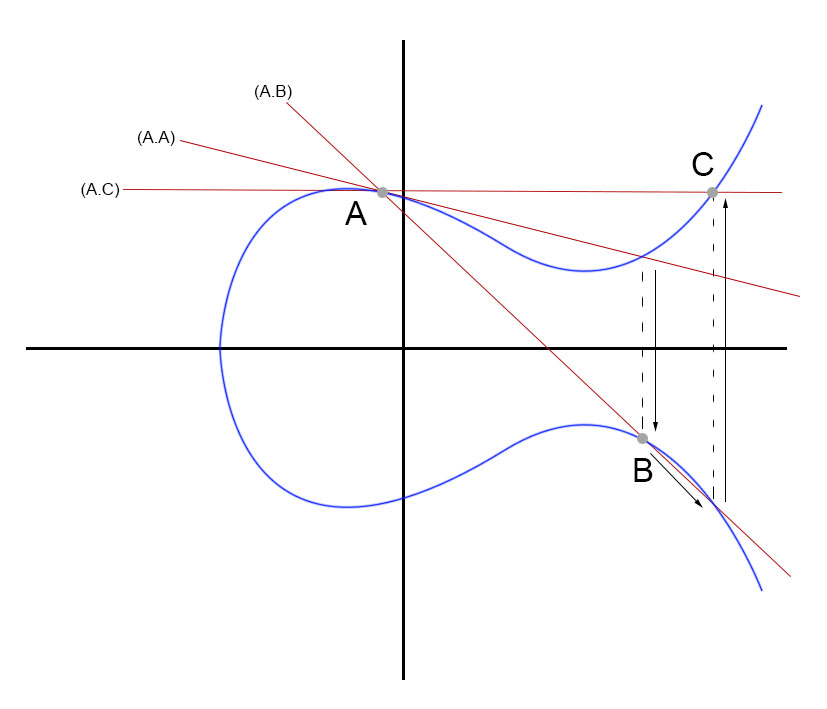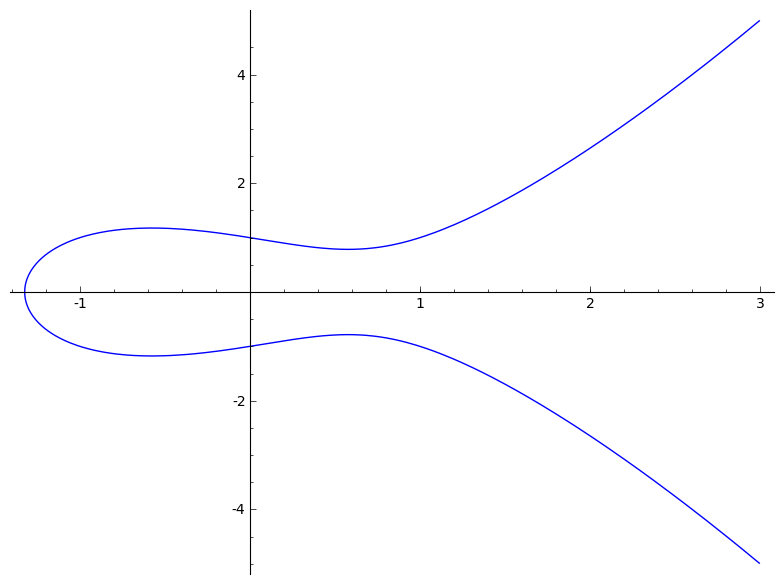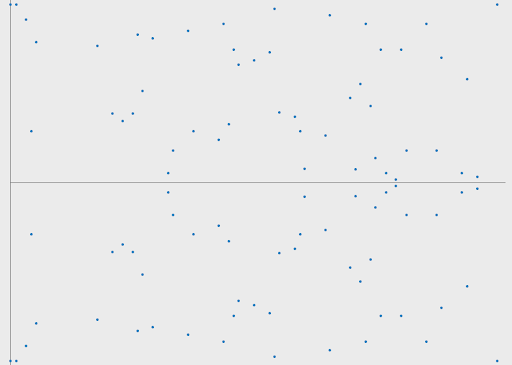# Engineering

## A brief investigation into elliptic curve cryptography

May 18th, 2020

An odd phenomenon I’ve observed – when I come across a piece of terminology for the very first time, I find myself hearing it again numerous times over the next couple of weeks. Perhaps my brain “tunes in” to it, and my inner chimp becomes alert to the strange new combination of words? Well whatever it is, that’s what happened to me recently with the term “elliptic curve cryptography”.

Now, I know (more or less) what elliptic curves are, and I know what cryptography is, but the two together? I wasn’t sure, so I decided to investigate: to find out what it is and why it’s useful – and then to write that up here.

## Cryptography

I’ll start with a quick refresher on cryptography. We can divide the field into two basic kinds of cryptography:

1. Symmetric cryptography
2. Asymmetric cryptography

Symmetric cryptography uses a single key which is shared among recipients of the encrypted message, allowing each to read it. One of the problems with symmetric cryptography relates to the secure distribution of the key among intended recipients.

Asymmetric cryptography, by contrast, uses a pair of keys – a public key, distributed openly, and a private key, kept secret by the sender.

We can think of these two methods in terms of physical keys and padlocks:

1. In symmetric cryptography, we put a padlock on our message and send the padlocked message to the recipient, and we separately send them a key to the padlock.
2. In asymmetric cryptography, the recipient sends us their padlock, and we send the message back safely locked up with that padlock.  We can go further though, and send our padlock back with theirs so that they know it’s us who send it (an odd thing to do in real life, perhaps, but this is only an analogy!) The important point is that the key to open the padlock never leaves the recipient, solving the problem of safe key distribution.

For the purposes of this article, we’ll focus on asymmetric cryptography. Let’s examine what this “padlock” is that the sender gives us. Rather than being a physical object made of steel, it’s a mathematical function. Think of a padlock: easy to close, hard to open (without the key). In cryptography, we use a mathematical function to close or lock the message – a function that is easy to do for the locking part, but hard to “unlock” without some special knowledge, that is, the key. These functions are often called “trapdoor” functions: easy to fall in to, hard to get out of – but personally I prefer the padlock analogy.

The most common type of trapdoor function used in cryptography (for example, in the RSA cryptosystem) is a simple product of primes. Take the number 33. It is the product of two primes, 3 and 11. For a low number like 33, that’s an easy operation to reverse, i.e., to find the prime factors – programmatically, we can step through the set of primes less than half 33 until we find two that give us the required product, a method known as a factor tree. However, it gets much harder to use a programmatic method to find the prime factors as the product gets large. There is a broadly linear relationship between the number x and the number of primes less than x (a number calculated with a function called the π function) – here is a graph showing the number of primes less than 1,000,000:Side note: while this relationship looks approximately linear, the distribution of primes seems to be random, and understanding the distribution of the primes is one of the most fascinating and enduring problems in mathematics.

Back to asymmetric cryptography: the “padlock” consists of the product of two large prime numbers, and the key consists of those two prime numbers. How big are those two large prime numbers? Well, in RSA, if our public key will be 2048 bits in length (a good length: it’ll take an attacker a long time to factor that into two primes), then the two prime factors will be roughly half that in length, which means roughly between 150 and 600 digits each. How many primes are there in this range? A lot – here is a handy tool using the Pi function to show you the number of primes under a given number. Note that this handy tool won’t let you request the Pi function for a number greater than 1.0e13 (10,000,000,000,000), for which there are roughly 346 billion primes, and that’s only for a 14-digit number. You can imagine therefore that there is a vast number of primes between 150 and 600 digits in length, and that lies at the heart of RSA’s security: it’s just very hard for a computer identify these prime factors quickly.

## Elliptic Curves

So what are elliptic curves, and what have they got to do with cryptography? Well, quite simply, an elliptic curve is:an elliptic curve is a type of cubic curve whose solutions are confined to a region of space that is topologically equivalent to a torus.

Got that?

OK, a simpler explanation is to say that an elliptic curve is the curve (the graph) that is produced by the points satisfying an equation. Specifically, an equation looking something like this:

y2 = x3 + ax + b

The graphs produced by equations like that look like this:There are two properties of these curves that are of special interest to us:

1. The curve has horizontal symmetry (reflecting over the x-axis)
2. Any non-vertical line will intersect the curve in at most three places.

Using these two properties, we can play a kind of game, as follows (visualised further down to make it easier to see what’s going on):

1. Pick a point on the curve above the x-axis, point A, and draw the line that is the tangent of the curve at A.
2. This line will intersect the curve at (at least) one other point. From that first point of intersection, draw a line vertically across the x-axis to intersect the mirror-image of that point below the x-axis. This is point B.
3. Draw another line through point A and B; this line will again intersect the curve below the x-axis.
4. As in step 2, reflect that intersection point above the x-axis, to give a new point, C.
5. We can continue on indefinitely (starting with a line through A and C, producing after reflection a new point D), and generate any number of new points by repeatedly drawing lines through previous points and reflecting around the x-axis.

We can notate this way of generating the points as follows, with a dot representing the function or process of drawing a line and reflecting:

A . A = B
A . B = C
A . C = D
A . D = E

I have illustrated this process on the diagram below, showing how we arrive at points B and C from A:We can repeat this process n times, arriving at a final point, which we can call X. If I give you X, could you find out n, even if you know what curve I am using, and what my starting point (A) was?

Side note: it would appear that to repeat the operation n times, I have to do n calculations to find X. However, it turns out that  there’s a shortcut (explained here) meaning that for a 256-bit integer (itself a vast number! In binary, think of 1 followed by 255 1’s or 0’s, the largest of which – all 1’s – corresponds to about 1×1077), we have at most 510 steps in our calculation. It is therefore a very efficient process.

However, even given the curve, the initial pair of points, and that final point, it is very hard to find out n. There is no known algorithm (such as the convenient “maximum 510 steps” method described above) to find n, therefore you must reverse the process step by step until you arrive back at `A . A = B`. If we had picked a 256-bit number consisting of 256 1’s, you would have to do all 1×1077 steps. Infeasible!

This should sound familiar: it’s like our padlock or trapdoor analogy, a function that is easy to do, but hard to undo. We can think of our padlock or public key as our initial point `(x, y)` or A, and our private key as the number n.

There is one added piece of complexity to the maths involved here. What if we end up, in one of our steps, with an x or y coordinate that cannot be stored given the fixed size of our public key? That is, if we have a 512-bit public key, and the length of x and y combined is larger than 512 bits, then either (or both) of x or y is too long.

You might be familiar with the modulo operation in arithmetic: it returns the remainder after division of one number by another. This same operation can be used in a curve to effectively “wrap” the curve within bounds; if x crosses a limit, it starts again at zero.

A useful way to visualise modular arithmetic is to think of a clock face, divided into 12 segments. Starting from 0, we can arrive at 1 by moving 1 segments, by 13 segments, 25, 37, 49 segments… There is a “wrapping” effect, and it’s just the same using a modulo operation on an elliptic curve, as follows:

y2 mod p = (x3 + ax + b) mod p

Here, p (being 1 greater than the maximum desirable value of x or y) is a large prime number; for a 512-bit key, it would be the largest prime number under 256 bits, ensuring that neither x nor y can be greater than 256 bits in length.

It’s also important to note that we only use the integer values along the curve, and not the infinitely many points between. Given that, and the modulo operation, our elliptic curve when graphed no longer resembles what we might expect a curve to look like – but note that it retains a horizontal axis of symmetry!

Here is the graph of `y2 = x3 - x + 1`:And here is the graph of that curve using a modulo of (the prime number) 97, integers only, that is,

y2 mod 97 = (x3 – x + 1) mod 97### A new trapdoor function

The essential point of this explanation of elliptic curves is to say that we’ve found another trapdoor function. How does it compare to the prime factor trapdoor function, and why is it useful?

## Why is it useful?

The answer to this question relates to how we can use elliptic curves to encrypt a message. Let’s pick a 256-bit integer for our n. We can run this through our “dot” function relating to a specific, pre-agreed elliptic curve to generate our encrypted message. The calculations involved in going through these n iterations is simple and fast for a computer to do (remember the shortcut mentioned above?), and therefore uses relatively little power.

By comparison, using RSA, to achieve the same level of security (as measured by the computing power required to reverse the trapdoor function), we require a 3072-bit integer (the product of our two secret primes), and we have to ask our computer to do its mathematical magic with that large integer, using correspondingly more compute power.

This table shows a comparison between RSA / DSA key size and the equivalent elliptic curve cryptography key size:

 RSA / DSA key size (bits) ECC key size (bits) 1024 160 2048 224 3072 256 7680 384 15360 512

(Source – NIST SP 800-57 Part 1 Rev. 3: a very detailed read! My table comes from page 64 of the PDF).

### Where is it used?

Elliptic curve cryptography is used when the speed and efficiency of calculations is of the essence. This is particularly the case on mobile devices, where excessive calculation will have an impact on the battery life of the device. Using a 256-bit key instead of a 3072-bit key for an equivalent level of security offers a significant saving. Similarly, less data needs to be transferred between parties to an encrypted message (for example, from server to client in an SSL handshake), and data bandwidth is another commonly constraining factor in mobile devices.

Therefore it’s no surprise to find that the Secure Enclave on Apple devices stores only elliptic curve private keys (256-bit).

By contrast, the AndroidKeyStore only supports encryption and decryption with RSA keys, as of Android 10 (the current version at the time of writing). There’s an interesting post on StackOverflow from someone claiming to be the Android engineer at Google with responsibility for AndroidKeyStore:

“For what it’s worth, I’m the Google engineer who owns AndroidKeyStore. I’ve been planning to add ECDH* support for a few years now, but it’s always been pre-empted by other features that were considered higher priority. I will get to it, though.”

* ECDH = Elliptic curve Diffie-Hellman, a key exchange protocol using elliptic curve cryptography.

Another notable use of elliptic curve cryptography is found in the blockchain technology used in cryptocurrencies, for example in both Ethereum and Bitcoin. The specific elliptic curve used by both currencies is called secp256k1, and the curve’s equation is:

y2 = x3 + 7

The starting point (equivalent to point A in our example above) used by secp256k1 is:

`x: 55066263022277343669578718895168534326250603453777594175500187360389116729240`

`y: 32670510020758816978083085130507043184471273380659243275938904335757337482424`

An interesting discussion of this particular elliptic curve and how it can be graphically represented can be found on the Bitcoin StackExchange.

Elliptic curve cryptography algorithms are available on cloud platforms too, for example in the AWS Key Management Service, and one of the use-cases suggested relates to cryptocurrencies; secp256k1 is supported, naturally. This service is in turn used by AWS itself in AWS Secrets Manager.

Other examples of the widespread use of elliptic curve cryptography, taking advantage of the benefits described above, include IoT devices (especially where power is a very limited resource), smart meters, RFID chips, and embedded systems. Hardware encryption modules such as the Cerberus Elliptic Curve Accelerator are available too.

There are some disadvantages to ECC, and it would be an oversight not to mention them. These include:

• As a relative newcomer to commercial cryptography, there are some outstanding patent problems with ECC.
• For the same reason, the standards are arguably less mature or well evolved than for RSA.
• There are new algorithms within ECC which may have unknown theoretical weaknesses (binary fields used, for even greater efficiency, instead of prime fields – beyond the scope of this article but there’s a starting point here).
• At least one backdoor in an ECC algorithm has emerged (DUAL_EC_DRBG), which while it can be avoided, raises concerns about other algorithms, especially if flawed random number generators are used.
• RSA is essentially easier to understand than ECC.
• As it is longer established, RSA is more widely deployed and therefore has generally better industry support.

## Final thoughts

I’ll finish by returning briefly to symmetric cryptography, which we left behind at the start of the article. The main disadvantage of asymmetric cryptography is that, compared to symmetric algorithms such as AES, it is slower and requires more data bandwidth (although as I’ve discussed, this is less the case with elliptic curve cryptography). What if we could use both types of cryptography, taking the advantages of each without the disadvantages?

That’s exactly what we can do: exchange keys using asymmetric cryptography such as ECC, and then once our keys have been safely shared, we can communicate using symmetric cryptography such as AES for more rapid and efficient encryption and decryption. This principle is used in SSL/TLS communication. A session key is negotiated using an asymmetric algorithm (using an ECC algorithm such as ECDH, briefly mentioned above, or with a non-ECC asymmetric algorithm such as RSA), and then that session key is used symmetrically for the duration of the connection. Once we are sure that both sides have securely shared a key, we can use the key with confidence. The advantage of using ECC in this case is to make the initial key-sharing stage faster and more efficient than RSA, and removes the need for both computers to have existing pre-shared keys.

A final advantage of elliptic curve cryptography is perhaps not immediately obvious: that is, it is an alternative to RSA and DSA. It may be that someone finds a way to predict the distribution of primes (perhaps by proving the Riemann hypothesis, one of the great outstanding problems in mathematics), and therefore make the problem of finding prime factors much easier to solve – or perhaps quantum computers will similarly offer a faster method. If that happens, then elliptic curve cryptography may be the best alternative, especially if a new weakness in RSA requires much larger keys.